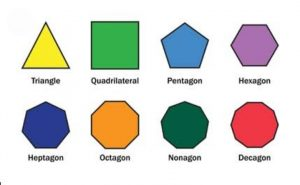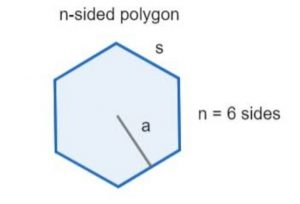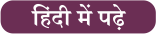Latest SSC jobs   »   Regular Polygons   »   Regular Polygons

# Regular Polygon – Definition, Formula, Area and Shapes

## Regular Polygons

A regular polygon is a two-dimensional plane closed figure made up of straight lines. It is made up of finite numbers of straight lines joined together to form a vertex and angles are also formed. If a polygon is made up of all straight lines having equal length then it is called a regular polygon while the polygons have different sides and angles called irregular polygons. In our daily life, we observe many polygon-shaped objects for various purposes. Examples of polygons are triangle, quadrilateral, pentagon, hexagon, octagon, etc. The regular polygons can be seen in the picture shown below.## What is Regular Polygon?

The polygons having all the sides and internal angles are equal then it is called a regular polygon. It is formed as the same length of straight lines and the angles formed by straight lines are also the same measure. Examples of regular polygons are equilateral triangles, squares, rhombus, etc.

## Regular Polygon Definition

A regular polygon is a closed figure having it’s all its sides and angles equal. It is a two-dimensional closed figure that lies with finite numbers of straight lines of equal length. The lines join at a point called vertex or corner and form an angle also there. You can see the regular polygons in the above figure.

## Regular Polygon Formula

As we already discussed a regular polygon, as it is is a two-dimensional closed figure having finite straight lines. It is made up of straight lines joining with each other. The formula used in a regular polygon is listed here.

The formula for finding the Sum of Interior Angles of a regular polygon

For a regular ‘n’ sided polygon, the sum of interior angles of a polygon is 180°(n-2)

The formula for finding the number of diagonals of a regular polygon

The number of diagonals of an “n-sided” polygon = [n(n-3)]/2

The formula for finding Each Interior Angles of a regular polygon

The measure of each interior angle of a regular n-sided polygon = [(n-2)180°]/n

The formula for finding Exterior Angle to a regular polygon

The measure of exterior angles of a regular n-sided polygon  = 360°/n

The formula for finding the area of a regular polygon

Area of regular polygon = (number of sides × length of one side × apothem)/2, where, the length of apothem is given as the

A = l²n/4 tan(π/l)

## Regular Polygons Interior Angles

As we know angle is formed by joining the two rays at a single point. In a regular polygon, all the sides and angles are equal so an equal number of angles as sides are formed. An interior angle is defined as the angle formed by two adjacent sides inside the figure. The interior angles are formed with sides joining at a point in the figure. The interior angles are measured in degrees or radians.

## Sum of Interior Angles of a Polygon

The sum of internal angles of a polygon is different for different figures. It is a constant value and can be determined easily. The formula to calculate the sum of interior angles of a polygon is mentioned here.

### Sum of the Interior Angles of a Polygon = 180 (n-2) degrees

Where n = number of sides of the polygon

 Polygon shapes Number of Interior Angles Sum of Interior Angles = (n-2) x 180° Triangle 3 180° Quadrilateral 4 360° Pentagon 5 540° Hexagon 6 720° Septagon 7 900° Octagon 8 1080° Nonagon 9 1260° Decagon 10 1440°

## Area of Regular Polygon

A regular polygon has equal sides and angles. The area of a regular polygon is the total region enclosed inside the closed figure. To find the area of a regular polygon an apothem is used sometimes. An apothem is defined as the line segment that joins the polygon’s center to the midpoint of any side and it is perpendicular to that side. All the vertices of a regular polygon lie on a circle passing inside the regular polygon or are known as an inscribed circle that makes a tangent at the midpoint of each side. So a regular polygon is also known as a tangential polygon. A regular polygon having n sides are shown in the below figure.The formula to calculate the area of a regular polygon is shown here

A = l²n/4 tan(π/l)

Where l = length of the side of the polygon

and, n = number of sides

## Regular Polygon Shapes

A regular polygon is a two-dimensional closed figure having all sides and angles equal. The regular polygon has infinite straight lines. The shapes of regular polygons are shown in the above figure. It has many shapes like triangle, square, rhombus, pentagon, hexagon, septagon, octagon, nonagon, decagon, etc.

### Regular Polygons: FAQs

Que.1 Define regular polygons?

Ans – The regular polygons are the shapes having equal lines and angles. It is a two-dimensional closed figure made with finite straight lines.

Que.2 What is another?

Ans – Apothem is defined as the line segment that joins the polygon’s center to the midpoint of any side and it is perpendicular to that side.

Que.3 Is the cube a regular polygon?

Ans – No, the cube is a three-dimensional figure having all sides equal but a regular polygon is only a 2d plane shape.

Que.4 Define the term interior angles of a regular polygon?

Ans – The interior angles of a regular polygon is the angle formed inside the figure having equal measure.

Que.5 What is the area of a regular polygon?

Ans – The area an on f regular polygon is the region covered inside the figure and it is calculated easily using the formula A = l²n/4 tan(π/l)#### Congratulations!General Awareness & Science Capsule PDF